Next: Time-varying coefficients Up: Designing filters Previous: Stretching the unit circle   Contents   Index

## Butterworth band-pass filter

We can apply the transformationto convert the Butterworth filter into a high-quality band-pass filter with center frequency. A further transformation can then be applied to shift the center frequency to any desired valuebetween 0 and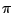. The transformation will be of the form,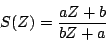where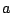andare real numbers and not both are zero. This is a particular case of the general form given above for unit-circle-preserving rational functions. We haveand, and the top and bottom halves of the unit circle are transformed symmetrically (ifgoes tothen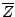goes to). The qualitative effect of the transformationis to squash points of the unit circle towardor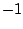.

In particular, given a desired center frequency, we wish to chooseso that:If we leave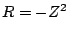as before, and let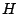be the transfer function for a low-pass Butterworth filter, then the combined filter with transfer function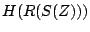will be a band-pass filter with center frequency. Solving forandgives:The new transfer function,, will have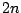poles andzeros (ifis the degree of the Butterworth filter).

Knowing the transfer function is good, but even better is knowing the locations of all the poles and zeros of the new filter, which we need to be able to compute it using elementary filters. Ifis a pole of the transfer function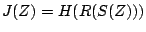, that is, if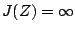, thenmust be a pole of. The same goes for zeros. To find a pole or zero ofwe set, whereis a pole or zero of, and solve for. This gives:(Hereandare as given above and we have used the fact that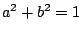). A sample pole-zero plot and frequency response ofare shown in Figure 8.20.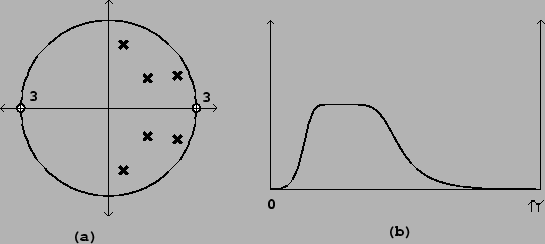Next: Time-varying coefficients Up: Designing filters Previous: Stretching the unit circle   Contents   Index
Miller Puckette 2006-12-30